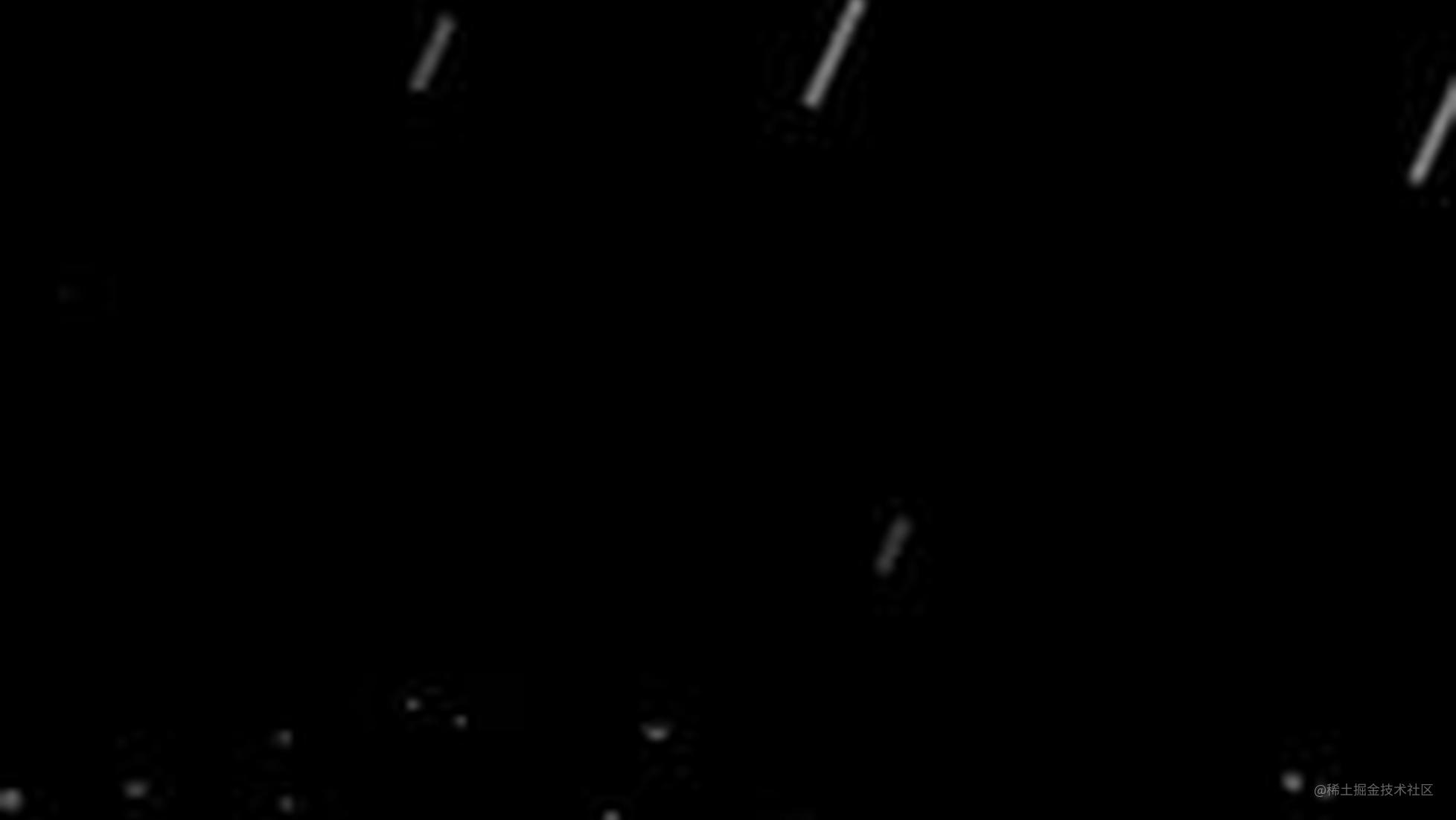# canvas实现 漂亮的下雨效果

### 解释

1、雨滴下落效果，移动鼠标控制下落方向
2、雨滴下落散成小水珠，小水珠的移动方向和鼠标移动方向相同
3、雨滴下落到鼠标坐标一定范围内，散成小水珠，同样的，小水珠的移动方向也和鼠标移动方向相同

#### 1、雨滴下落效果，移动鼠标控制下落方向

speed 是一个固定的值，表示雨滴下落速度，
speedx 是一个和鼠标移动方向有关系的变量，
`speedx = speedx + (maxspeedx - speedx) / 50`

`maxspeedx = (e.clientX - canvasEl.clientWidth / 2) / (canvasEl.clientWidth / 2)`
e.clientX：鼠标距离可视区域左边的值
canvasEl.clientWidth：整个可视区域的宽度

maxspeedx 的取值范围是 -1 到 1，他的值越接近 -1，说明方向越向左，值越接近1，说明方向越向右。

speed 和上面x坐标中提到的一样，是一个固定值，表示雨滴下落速度，

`x坐标 = 雨滴x坐标的值 + 雨滴长度 * speedx`
`y坐标 = 雨滴y坐标的值 + 雨滴长度`

#### 2、雨滴下落散成小水珠，小水珠的移动方向和鼠标移动方向相同

`小水珠x坐标： 删除的雨滴x坐标 + 删除的雨滴长度 * speedx`
`小水珠y坐标：删除的雨滴y坐标 + 删除的雨滴长度`

`vx = vx + speedx / 2`
`小水珠的x坐标 =原x坐标 + vx`

speedx：上面提到的和鼠标移动方向相关的一个变量，这里的作用就是用来控制小水珠的移动方向和其他方向相同
`speedx / 2`，除2是为了使 让小水珠 在x轴的移动距离短一点，看上去更真实点

`vy = vy + gravity`
`小水珠的y坐标 = 原y坐标 + vy;`
vy：一个负数
gravity：重力，一个正数，完整代码里设置的是0.5

#### 3、雨滴下落到鼠标坐标一定范围内，散成小水珠，同样的，小水珠的移动方向也和鼠标移动方向相同

`AB = Math.sqrt(BC*BC + AC * AC)`

BC = 雨滴x坐标 - 鼠标x坐标
AC = 雨滴y坐标 - 鼠标y坐标
Math.sqrt()方法用来计算一个数的平方根

### 完整代码

``````<!doctype html>
<html lang="en">
<meta charset="UTF-8">
<style>
* {
margin: 0;
}
</style>
<body>
<canvas id="canvas" style="position: absolute; height: 100%; width:100%;"></canvas>
<script>
function main() {
// 获取canvas元素
var canvasEl = document.getElementById('canvas');
var ctx = canvasEl.getContext('2d');
// canvas画布的 背景颜色
var backgroundColor = '#000';

// canvas画布的宽 等于 可视区域的宽
canvasEl.width = canvasEl.clientWidth;
// canvas画布的高 等于 可视区域的高
canvasEl.height = canvasEl.clientHeight;

// 保存小水珠的数组
// 雨滴下落后散成小水珠，小水珠就是一些圆弧
var dropList = [];

// 重力
// 雨滴下落后散成小水珠，小水珠会先上升后下降，主要是因为 gravity 这个变量的缘故
var gravity = 0.5;

// 保存雨滴的数组
// 每个雨滴 都是 画的一条线
var linelist = [];

// 保存鼠标的坐标
// mousePos 代表x轴的值，mousePos 代表y轴的值
var mousePos = [0, 0];

// 跟随鼠标， mouseDis 大小区域内的雨滴会消失，形成散落效果
// 以mousePos为圆心，mouseDis为半径，这个范围内的雨滴 都会散开，形成许多小水珠
var mouseDis = 35;

// 更新一次动画，画lineNum 条雨滴，lineNum 值越大，下雨就越密集
var lineNum = 3;

// 跟随鼠标方向 变化下雨方向的 速度
// 鼠标移动后，下雨的方向 会慢慢改变，主要靠speedx 这个变量
var speedx = 0;

// maxspeedx 为 speedx 可以取的最大值
// 当 speedx = maxspeedx时，下雨方向 会 随鼠标移动方向立即改变
var maxspeedx = 0;

// 页面大小发生变化时，重置canvas画布大小
window.onresize = function () {
canvasEl.width = canvasEl.clientWidth;
canvasEl.height = canvasEl.clientHeight;
}

//移动鼠标触发事件
window.onmousemove = function (e) {
//  设置mousePos 等于 鼠标坐标
//  e.clientX 为距离 浏览器窗口可视区域 左边的距离
//  e.clientY 为距离 浏览器窗口可视区域 上边的距离
mousePos = e.clientX;
mousePos = e.clientY;

// 通过鼠标位置，设置 maxspeedx的值，取值范围是 -1 到 1
// maxspeedx的值，关系到
// 1、雨滴的方向
// 2、雨滴下落的方向
// 3、雨滴下落方向 跟随 鼠标移动方向变化的速度
// 4、小水珠的移动方向
// 值越接近1，表示方向越向右
// 值越接近-1，表示方向越向左
maxspeedx = (e.clientX - canvasEl.clientWidth / 2) / (canvasEl.clientWidth / 2);
}

// 根据参数，返回一个rgb颜色，用于给雨滴设置颜色
function getRgb(r, g, b) {
return "rgb(" + r + "," + g + "," + b + ")";
}

// 画 一滴雨（一条线）
function createLine(e) {
// 随机生成 雨滴的长度
var temp = 0.25 * (50 + Math.random() * 100);
// 一个 line 对象，代表一个雨滴
var line = {
// 雨滴下落速度
speed: 5.5 * (Math.random() * 6 + 3),
// 判断是否删除，值为true就删除
die: false,
// 雨滴x坐标
posx: e,
// 雨滴y坐标
posy: -50,
// 雨滴的长度
h: temp,
// 雨滴的颜色
color: getRgb(Math.floor(temp * 255 / 75), Math.floor(temp * 255 / 75), Math.floor(temp * 255 / 75))
};
// 把创建好的line（雨滴）对象，添加到保存雨滴的数组
linelist.push(line);
}

// 画一个小水珠（雨滴散开后的小水珠就是一个个的圆弧）
function createDrop(x, y) {
// 一个 drop 对象，代表一个圆弧
var drop = {
// 判断是否删除，值为true就删除
die: false,
// 圆弧圆心的x坐标
posx: x,
// 圆弧圆心的y坐标
posy: y,
// vx 表示 x轴的值 变化的速度
vx: (Math.random() - 0.5) * 8,
// vy 表示 y轴的值 变化的速度 取值范围：-3 到 -9
vy: Math.random() * (-6) - 3,
// 圆弧的半径
radius: Math.random() * 1.5 + 1
};
return drop;
}

// 画一定数量的小水珠
// 随机生成一个数 maxi
// maxi 代表要画小水珠的数量
var maxi = Math.floor(Math.random() * 5 + 5);
for (var i = 0; i < maxi; i++) {
dropList.push(createDrop(x, y));
}
}

// 开始调用update函数，更新动画
window.requestAnimationFrame(update);
// 更新动画
function update() {
// 如果保存小水珠的数组有内容
if (dropList.length > 0) {
// 遍历保存小水珠的数组
dropList.forEach(function (e) {
//设置e.vx，vx表示x坐标变化的速度
// (speedx)/2 是为了，让小水珠 在x轴的移动距离短一点，看上去更真实点
// 也使 小水珠的移动方向 和 雨滴方向，雨滴下落方向，鼠标移动方向相同
e.vx = e.vx + (speedx / 2);
e.posx = e.posx + e.vx;

//设置e.vy，vy表示y坐标变化的速度
// e.vy的范围是-3 到 -9，而这时e.posy（y坐标）一定是正值，所以 e.posy的值会先减小后增大
// 也就是实现 雨滴散成小水珠，小水珠会先上升后下降的效果
e.vy = e.vy + gravity;
e.posy = e.posy + e.vy;

// 如果 小水珠y坐标 大于 可视区域的高度，设置die属性为true
// 小水珠如果超出可视区域就删除掉
if (e.posy > canvasEl.clientHeight) {
e.die = true;
}
});
}

// 删除 die属性为ture 的数组成员
// 可视区域外的小水珠删除掉
for (var i = dropList.length - 1; i >= 0; i--) {
if (dropList[i].die) {
dropList.splice(i, 1);
}
}

// 设置下雨方向变换的速度，取值范围： -1 到 1
// 当 speedx = maxspeedx时，下雨方向 会 随鼠标移动方向立即改变
speedx = speedx + (maxspeedx - speedx) / 50;

// 根据lineNum的值，画一定数量雨滴
for (var i = 0; i < lineNum; i++) {
// 调用createLine 函数，参数是雨滴x坐标
createLine(Math.random() * 2 * canvasEl.width - (0.5 * canvasEl.width));
}

// 设置结束线，也就是雨滴散开 形成许多小水珠的位置
var endLine = canvasEl.clientHeight - Math.random() * canvasEl.clientHeight / 5;

// 遍历保存雨滴的数组
linelist.forEach(function (e) {

// 利用勾股定理 确定一个范围，在这个范围内雨滴会散开形成小水珠
// e.posx + speedx * e.h 是雨滴x坐标
// e.posy + e.h 是雨滴y坐标
var dis = Math.sqrt(((e.posx + speedx * e.h) - mousePos) * ((e.posx + speedx * e.h) - mousePos) + (e.posy + e.h - mousePos) * (e.posy + e.h - mousePos));

// 如果在mouseDis区域内，就删除雨滴，画一些小水珠（圆弧）
// 实现鼠标碰到雨滴，雨滴散成小水珠的效果
if (dis < mouseDis) {
// 删除 雨滴
e.die = true;
// 画一些小水珠（圆弧）
madedrops(e.posx + speedx * e.h, e.posy + e.h);
}

// 如果雨滴超过 结束线，删除雨滴，画一些小水珠（圆弧）
if ((e.posy + e.h) > endLine) {
e.die = true;
madedrops(e.posx + speedx * e.h, e.posy + e.h);
}

// 如果 雨滴 y坐标 大于 可视区域的高度，设置die属性为true
// 如果 雨滴 超出可视区域就删除掉
if (e.posy >= canvasEl.clientHeight) {
e.die = true;
} else {
// 逐渐增加 雨滴 y坐标的值
e.posy = e.posy + e.speed;

// 变化雨滴 x坐标
// * speedx 用来控制雨滴 下落 方向
// 使 雨滴下落方向 和 鼠标移动方向相同
e.posx = e.posx + e.speed * speedx;
}
});

// 删除 die属性为ture 的数组成员
// 鼠标区域内的，超过结束线的，可视区域外的雨滴删除掉
for (var i = linelist.length - 1; i >= 0; i--) {
if (linelist[i].die) {
linelist.splice(i, 1);
}
}

// 渲染
render();
// 递归调用 update，实现动画效果
window.requestAnimationFrame(update);
}

// 渲染
function render() {
// 画一个和可视区域一样大的矩形
ctx.fillStyle = backgroundColor;
ctx.fillRect(0, 0, canvasEl.width, canvasEl.height);

// 画雨滴效果
ctx.lineWidth = 5;
linelist.forEach(function (line) {
ctx.strokeStyle = line.color;
ctx.beginPath();
ctx.moveTo(line.posx, line.posy);

// * speedx 用来控制雨滴方向
// 使 雨滴方向 和 鼠标移动方向相同
ctx.lineTo(line.posx + line.h * speedx, line.posy + line.h);
ctx.stroke();
});

// 画雨滴散开形成小水珠效果
ctx.lineWidth = 1;
ctx.strokeStyle = "#fff";
dropList.forEach(function (e) {
ctx.beginPath();
ctx.arc(e.posx, e.posy, e.radius, Math.random() * Math.PI * 2, 1 * Math.PI);
ctx.stroke();
});

// 解开注释，可看见鼠标范围
/*
ctx.beginPath();
ctx.arc(mousePos, mousePos, mouseDis, 0, 2 * Math.PI);
ctx.stroke();
*/
}
}
</script>
</body>

</html>